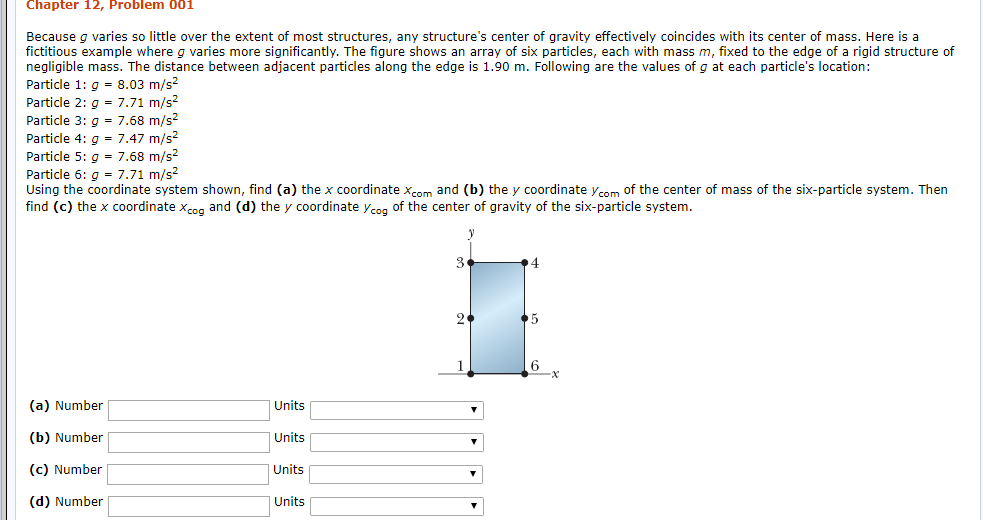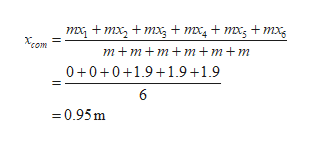# Chapter 12, Problem d01Because g varies so little over the extent of most structures, any structure's center of gravity effectively coincides with its center of mass. Here is afictitious example where g varies more significantly. The figure shows an array of six particles, each with mass m, fixed to the edge of a rigid structure ofnegligible mass. The distance between adjacent particles along the edge is 1.90 m. Following are the values of g at each particle's location:Particle 1: g =8.03 m/s2Particle 2: g = 7.71 m/s2Particle 3: g= 7,68 m/s2Particle 4: q 7,47 m/s2Particle 5: q= 7.68 m/s2Particle 6: g = 7.71 m/s2Using the coordinate system shown, find (a) the x coordinate xcom and (b) the y coordinate ycom of the center of mass of the six-particle system. Thenfind (c) the x coordinate x and (d) the y coordinate y of the center of gravity of the six-particle system4526(a) NumberUnits(b) NumberUnits(c) NumberUnits(d) NumberUnits

Question
244 viewshelp_outlineImage TranscriptioncloseChapter 12, Problem d01 Because g varies so little over the extent of most structures, any structure's center of gravity effectively coincides with its center of mass. Here is a fictitious example where g varies more significantly. The figure shows an array of six particles, each with mass m, fixed to the edge of a rigid structure of negligible mass. The distance between adjacent particles along the edge is 1.90 m. Following are the values of g at each particle's location: Particle 1: g =8.03 m/s2 Particle 2: g = 7.71 m/s2 Particle 3: g= 7,68 m/s2 Particle 4: q 7,47 m/s2 Particle 5: q= 7.68 m/s2 Particle 6: g = 7.71 m/s2 Using the coordinate system shown, find (a) the x coordinate xcom and (b) the y coordinate ycom of the center of mass of the six-particle system. Then find (c) the x coordinate x and (d) the y coordinate y of the center of gravity of the six-particle system 4 5 2 6 (a) Number Units (b) Number Units (c) Number Units (d) Number Units fullscreen
check_circle

Step 1

The edge length is given as 1.90m.let the coordinates can be represented as.

Step 2

The weight of six particles.

Step 3

(a)The value of xcom...help_outlineImage Transcriptioncloseтх, + тx, + тx,+ mx, t mxs + mхx, Xcom m+m+mm+m+m 0+0+0+1.91.9+1.9 6 0.95m fullscreen

### Want to see the full answer?

See Solution

#### Want to see this answer and more?

Solutions are written by subject experts who are available 24/7. Questions are typically answered within 1 hour.*

See Solution
*Response times may vary by subject and question.
Tagged in

### Physics